Study Materials:
CBSE NOTES ⇒ Class 10th ⇒ Science ⇒ 10. Light-Reflection and Refraction

## 10. Light-Reflection and Refraction

### Introduction

px;">

10. Light-Reflection and Refraction

Light makes things visible. The sun light helps us to see the objects during light.

How we able to see any object?

An object reflects light that falls on it. This reflected light, when received by our eyes, enables us to see things.

A ray of light: When light travels from its source of light, it looks like straight line, this line is called a ray of light.

Shadow: When a beam of light pass through an opaque object and get reflected and it form a shadow of that opaque object.

Diffraction of light : If an opaque object on the path of light becomes very small, light has a tendency to bend around it and not walk in a straight line – an effect known as the diffraction of light.

### Reflection Of Light:

When a ray of light falls on polished surface, it turns or reflects most of the light in the same medium from which it comes. This phenomenom is known as reflection of lightThe laws of reflection of light:

(i)   The angle of incidence is equal to the angle of reflection, and
(ii)  The incident ray, the normal to the mirror at the point of incidence and the reflected ray, all lie in the same plane.

These laws of reflection are applicable to all types of reflecting surfaces
including spherical surfaces.

Some common wonderful phenomena

There occur some common wonderful phenomena due to reflection of light. These are followings;

Image formation by mirrors, the twinkling of stars, the beautiful colours of a rainbow, bending of light by a medium.

Type of Reflection:

1. Specular or regular reflection: Such type of reflection takes place from shiny or polished surface and incident rays remain parallel to each other after reflection.2. Diffused or irregular reflection: Such type of reflection takes place from

rough surface and incident rays do not remain parallel to each other after

reflection.Mirror : This is a shiny and more polished reflecting surface, which make the image of an object kept front of it.

Types of Mirror:

There are two types of mirror.

(A)  Plane Mirror : Its reflecting surface is straight and flat.(B)  Spherical Mirror : It has curved reflecting surface. This curved reflecting surface can be curved inward or out outwart.

On the basis of this curvature, spherical mirror are two types.

(i) Concave Mirror : Its reflecting surface is inward.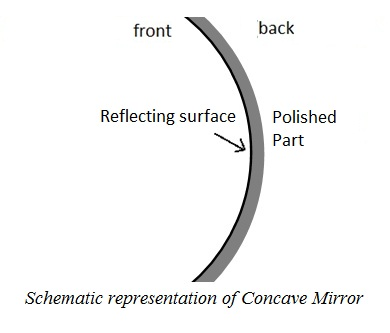(ii) Convex Mirror : Its reflecting surface is outward.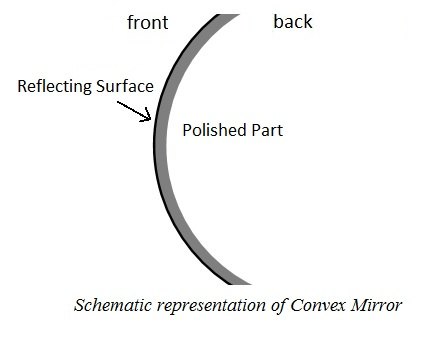The Parts Of Spherical Mirror :

(i) Pole : The centre of reflecting surface of spherical mirror is called its pole. It is denoted by English letter P.

(ii) The Centre of Curvature : The reflecting surface of a spherical mirror forms a part of a sphere. This sphere has a centre. This point is called the centre of curvature of the spherical mirror.

(iii) The Radius of Curvature : The distance between Pole of the spherical mirror and the center of curvature is known as the radius of curvature.

(iv) Principal Axis : The straight line passing through the pole and the centre of curvature of spherical mirror is known as principal axis.

(v) Principal Focus : There is an another point F on principal axis lie between half of the pole and the center of curvature which is called the principal focus. Incident rays parallel to the principal axis intersect at principal focus after reflection in concave mirror and it seems to intersect in convex mirror.

(vi) Focal Length : Distance between the pole and the principal focus of the mirror is called Focal Length. It is denoted by small 'f' of English alphabets. This distance is half of the radius of the curvature.

(vii) Aperture : The reflecting surface of a spherical mirror is by and large spherical. The surface, then, has a circular outline. The diameter of the reflecting surface of spherical mirror is called its aperture.

The Position, Nature and Size of Image:

The position of object: The place where an object is placed.

The position of Image : The place where mirror forms the image of an

object.

The Size of Image : This is the size of image which tells that the image of object is formed either smaller or larger than the object.

The nature of image : It is known by the nature of image that what type of image is formed by mirror like virtual and errect or real and inverted.

There are two types of nature of images.

(i) Real and inverted : This types of images always form infront of mirror.

(ii) Virtual and errect : This types of images always form behind the mirror.

Image Formation by Spherical Mirrors:

The formation of image in concave mirror is depended on the position of  an object. An object placed between the pole (P) and principal focus (F) form only virtual and errect image otherwise all others images are real  and inverted that kept any other places before the concave mirror.

Some positions, natures and sizes of images formed by concave mirror.

• The image of an object placed at infinity is real and inverted and very small like a point size and Image forms at Focus.
• The Image of an object placed far away from centre of curvature C forms real and inverted and very small image between Focus and centre of curvature.
• The Image of an object placed at Centre of curvature (C) forms real and inverted at C and equal in size of object.
• The image of an object placed between centre of curvature and principal focus forms real and inverted, far aways from C and magnified.
• The image of an object placed at principal focus forms real and inverted and very large to object at infinity.
• The image is virtual and errect, larger than object and behind the mirror when object placed between P and F.

Use Of Concave Mirror:

(i)    Concave mirrors are commonly used in torches, search-lights and vehicles headlights to get powerful parallel beams of light.

(ii)    They are often used as shaving mirrors to see a larger image of the face.

(iii)   Concave mirrors are used to see large images of the teeth of patients by the dentists.

(iv)   Large concave mirrors are used to concentrate sunlight to produce  heat in solar furnaces.

Image Formation By Convex mirror:

The image formed by convex mirror is always virtual and errect and smaller than the object.

Use Of Convex Mirror:

(i)   Convex mirrors are commonly used as rear-view (wing) mirrors in vehicles.

(ii)   It is used as reflector for street lighting purposes.

(iii)   Convex mirrors are useful for inspecting places difficult to get to.

(iv)   convex mirrors are also used for security situations.

Sign Convention for Reflection by Spherical Mirrors:

Reflection of light by spherical mirrors form a cartesian plane which is a

set of sign conventions called the New Cartesian sign Convention.

These are as follows -

• The pole (P) of the mirror is taken as the origin.
• The principal axis of the mirror is taken as the x-axis (X’X) of the
coordinate system.
• The object is always placed to the left of the mirror. This implies
that the light from the object falls on the mirror from the left-hand
side.
• All distances parallel to the principal axis are measured from the
pole of the mirror.
• All the distances measured to the right of the origin (along
+ x-axis) are taken as positive while those measured to the left of
the origin (along – x-axis) are taken as negative.
• Distances measured perpendicular to and above the principal axis
(along + y-axis) are taken as positive.
• Distances measured perpendicular to and below the principal axis
(along –y-axis) are taken as negative.

Mirror Formula: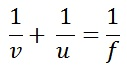Uses of Mirror formula:

Example 1: An object is placed 20 cm in front of a concave mirror of focal length 15 cm. Find the position of object, nature and size of image.

Solution:

Object-distance (u) = - 20 cm [ Distance of object is always negative (-) ]

focal length (f) = - 15 cm [Focal length in concave mirror is negative (-) ]

Image-Distance (v) = ?

Using mirror formula;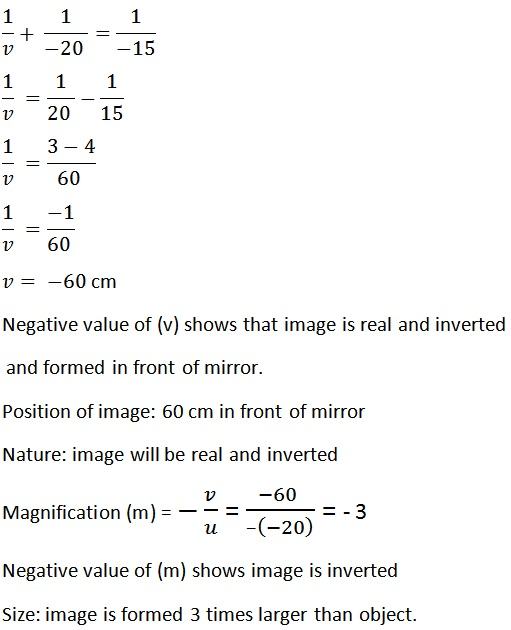Example 2. An object of height 5 cm is placed 15 cm away from a convex mirror of focal length 15 cm. Find the position, nature and size of image formed.

Solution:

Object-distance (u) = -15 cm

Focal length (f) = 15 cm       Focal length in convex mirror is positive (+) ]

Image-distance (v) = ?

Using mirror formula:Position of image: 7.5 cm back of mirror

Nature of image: Vertual and irect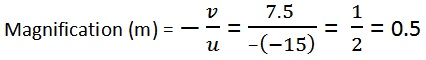Size: Half of the object (smaller).

Other Pages of this Chapter:

Important Study materials for classes 06, 07, 08,09,10, 11 and 12. Like CBSE Notes, Notes for Science, Notes for maths, Notes for Social Science, Notes for Accountancy, Notes for Economics, Notes for political Science, Noes for History, Notes For Bussiness Study, Physical Educations, Sample Papers, Test Papers, Mock Test Papers, Support Materials and Books.Mathematics Class - 11th

NCERT Maths book for CBSE Students.

books

## Study Materials List:

##### CBSE NOTES ⇒ Class 10th ⇒ Science
1. Chemical Reactions and Equations
2. Acids, Bases and Salts
3. Metals and Non-metals
4. Carbon and its Compounds
5. Periodic Classification of Elements
6. Life Processes
7. Control and Coordination
8. How do Organisms Reproduce
9. Heredity and Evolution
10. Light-Reflection and Refraction
11. Human Eye and Colourful World
12. Electricity
13. Magnetic Effects of Electric Current
14. Sources of Energy
15. Our Environment
16. Management of Natural Resources

New Books

See More ...